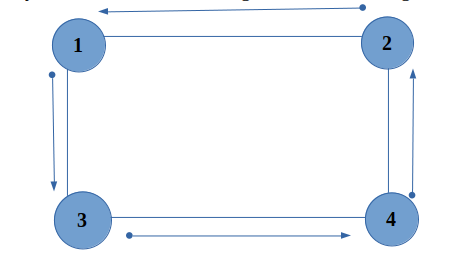## Checking a undirected graph for acyclicity and finding a cycle

We have been given an undirected graph with n nodes and m edges. We are required to check whether the given graph contains cycle or not. If the graph is cyclic then print any one cycle in the graph.

A graph is called cyclic if there is a path in the graph which starts from a vertex and ends at the same vertex with non-repeating edges. That path is called a cycle. An acyclic graph is a graph which has no cycle.Algorithm used:-

We can solve this problem using Depth First Search (DFS).

1. Detecting cycles using DFS :-

• We will be doing a series of DFS to solve this problem.

• Initially we mark all the nodes as unvisited i.e. vis[i]=0 for all (1<=i<=n)

• If a node (let’s say x) is unvisited then we will perform DFS over that particular node and mark that node as partially visited i.e. vis[x] = 1 while entering.

• While exiting from that node we will mark that node as completely visited i.e. vis[x] =2.

• Now if while DFS of any node we find a vertex which is partially visited, then this implies that we have found a cycle.

• In simple words, let’s suppose we start the DFS from 1 which is firstly marked as partially visited and node 2 is called recursively. In the same way node 2 is marked partially visited and node 3 is called recursively and the same goes for node 4 also. Now during the DFS of node 4 node 1 is called which is already marked as partially visited. So this implies that a cycle is found which is starting at node 1 and ending at node 4.• Time complexity of this algorithm : O(N+M)

• where N -> No of nodes in the graph

• M -> No of edges in the graph

Implementation :-

Input :-
6 9
1 2
3 1
1 4
5 1
3 5
6 3
4 5
5 6
2 3

Output :-
Cycle found : 3 2 1

Input :-
5 4
4 1
3 5
2 1
3 2

Output :-
Acyclic Graph

Practice Problems :-

It is highly recommended to solve below problems, they are handpicked by Programmers Army: